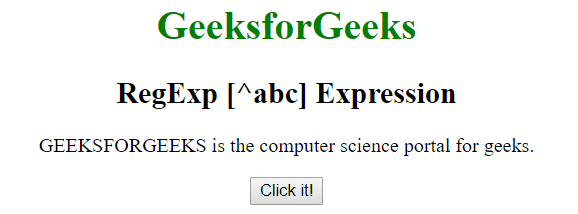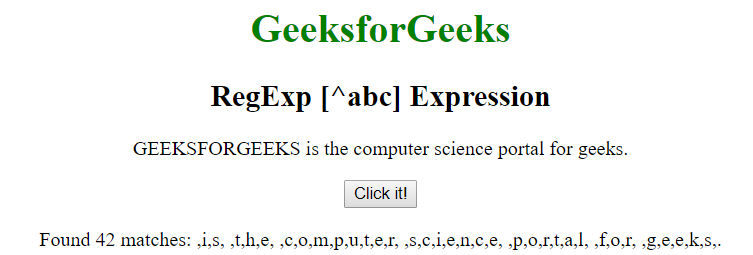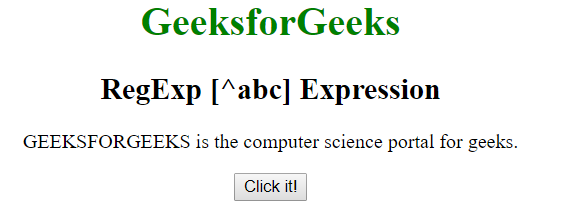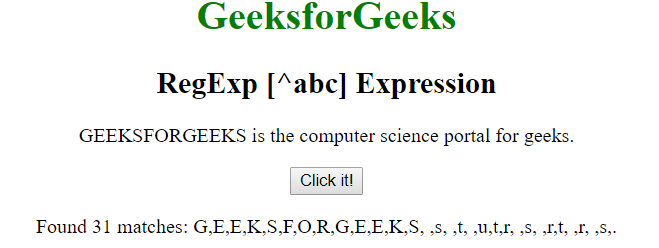# JavaScript | RegExp [^abc] Expression

• Last Updated : 22 Apr, 2019

The RegExp [^abc] Expression in JavaScript is used to search any character which is not between the brackets. The character inside the brackets can be a single character or a span of characters.

• [A-Z]: It is used to match any character from uppercase A to uppercase Z.
• [a-z]: It is used to match any character from lowercase a to lowercase z.
• [A-z]: It is used to match any character from uppercase A to lowercase z.
• [abc…]: It is used to match any character between the brackets.

Syntax:

`/[^abc]/ `

or

`new RegExp("[^abc]")`

Syntax with modifiers:

`/\[^abc]/g `

or

`new RegExp("[^abc]", "g")`

Example 1: This example searches the characters which are not present between [A-G] i.e uppercase A to uppercase G in the whole string.

 ```<``html``>`` ` `<``head``>``    ``<``title``>``        ``JavaScript RegExp [^abc] Expression``    `````` ` `<``body` `style``=``"text-align:center"``>`` ` `    ``<``h1` `style``=``"color:green"``>``        ``GeeksforGeeks``    ````     ` `    ``<``h2``>RegExp [^abc] Expression``     ` `    ``<``p``>``        ``GEEKSFORGEEKS is the computer ``        ``science portal for geeks.``    ````     ` `    ``<``button` `onclick``=``"geek()"``>``        ``Click it!``    ````     ` `    ``<``p` `id``=``"app"``>``     ` `    ``<``script``>``        ``function geek() {``            ``var str1 = "GEEKSFORGEEKS is the computer "``                        ``+ "science portal for geeks.";``             ` `            ``var regex4 = /[^A-Z]/g;``            ``var match4 = str1.match(regex4);`` ` `            ``document.getElementById("app").innerHTML = ``                    ``"Found " + match4.length ``                    ``+ " matches: " + match4;``        ``}``    `````` ` `                    `

Output:

• Before Clicking the button:• After Clicking the button:Example 2: This example searches the characters which are not present between [a-g] i.e lowercase a to lowercase g in the whole string.

 ```<``html``>`` ` `<``head``>``    ``<``title``>``        ``JavaScript RegExp [^abc] Expression``    `````` ` `<``body` `style``=``"text-align:center"``>`` ` `    ``<``h1` `style``=``"color:green"``>``        ``GeeksforGeeks``    ````     ` `    ``<``h2``>RegExp [^abc] Expression``     ` `    ``<``p``>``        ``GEEKSFORGEEKS is the computer ``        ``science portal for geeks.``    ````     ` `    ``<``button` `onclick``=``"geek()"``>``        ``Click it!``    ````     ` `    ``<``p` `id``=``"app"``>``     ` `    ``<``script``>``        ``function geek() {``            ``var str1 = "GEEKSFORGEEKS is the computer "``                        ``+ "science portal for geeks.";``             ` `            ``var regex4 = /[^a-p]/g;``            ``var match4 = str1.match(regex4);`` ` `            ``document.getElementById("app").innerHTML = ``                        ``"Found " + match4.length``                        ``+ " matches: " + match4;``        ``}``    `````` ` `                    `

Output:

• Before Clicking the button:• After Clicking the button:Supported Browsers: The browsers supported by RegExp [^abc] Expression are listed below: